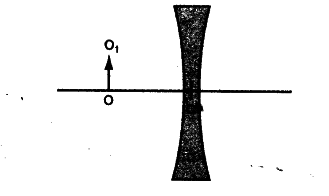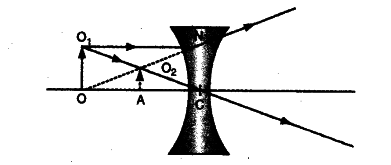# In figure given below

In figure given below OC = f, the focal length of the lens. Copy the diagram in your answer book. Draw rays from the linear object O\$O_{1} \$ and obtain the image formed by the lens.The ray diagram is as shown below :